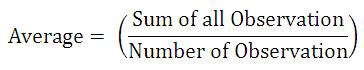# SSC CHSL Aptitude Study Material: Averages

the SSC experts of Jagranjosh.com have provided with the description of one of the most important chapters in quantitative aptitude – Average, to let you understand the basic concept of the topic.

Created On: Jan 2, 2018 19:54 ISTssc chsl study material

Staff Selection Commission conducts SSC Combined Higher Secondary Level Examination every year for recruitment of candidates for the posts of Data Entry Operator and Lower Division Clerk in various central government departments.

The SSC – CHSL Exam comprise one written paper that consists of 100 questions divided in 4 different sections -- General Intelligence, General Awareness, Quantitative Aptitude, and English Language.The Quantitative Aptitude Section of SSC - Combined Higher Secondary Level Examination contains 25 questions from topics like Percentage; Average; Profit, Loss & Discount; Simple Interest & Compound Interest; Simplification; Geometry; Trigonometry; etc. Though the quantitative aptitude is quite a challenging in nature, it is considered as the highly scoring section of SSC – CHSL Exam.

Here, the SSC experts of Jagranjosh.com have provided with the description of one of the most important chapters in quantitative aptitude – Average, to let you understand the basic concept of the topic.

Take Online Quiz

Averages

The term Average refers to the sum of all observations divided by the total number of observations. Average is used quite regular in our day to day life. For example to calculate the average marks of the students, Average height of a particular group etc. The term average is also referred to as ‘Mean’. Basic formula to calculate the average is as follows:Example: What is the average of First 10 Prime numbers?

Solution: First 10 Prime numbers are 2,3,5,7,11,13,17,19,23,29.

Hence, Average = {2+3+5+7+11+13+17+19+23+29} / 10
= 129 / 10
= 12.90

So, Average of First 10 Prime numbers is 12.90.

Example: The total number of sales visits made by a Salesman in the month of June is 90. What is the Average visit he makes per day?

Solution: Number of days in the month of June are 30

Hence, Average Visit per day = Number of total visits / Number of total days
= 90 / 30
= 3

So, the salesman makes 3 visits per day.

Question from Previous Year Question Paper:

1. The mean of 11 numbers is 35. If the mean of first 6 numbers is 32 and that of the last 6 numbers is 37, find the sixth number. (SSC Higher Secondary Exam 2012)

1. 28
2. 29
3. 30
4. 27

Solution:

Total value of 11 numbers = 11x35 = 385
Total value of first 6 numbers = 6x32 = 192
Total value of last 6 numbers = 6x37 = 222
Value of sixth number = (192+222) – 385 = 29.

Answer: 2

2. In a 20 over match, the required run rate to win is 7.2. If the run rate is 6 at the end of the 15th over, the required run rate to win the match is - (SSC Higher Secondary Exam 2012)

1. 1.2
2. 13.2
3. 10.8
4. 12

Solution:

Total runs required = 20x7.2 = 144
Runs made at the end of 15th over = 6x15 = 90
Required run rate to win = (144-90)/4 = 10.8

Answer: 3

3. The average weight of the 8 oarsmen is boat is increased by when one of the crew who weighs 60kg is replaced by a new man. The weight of the new man in kg is-. (SSC Higher Secondary Exam 2012)

1. 70
2. 68
3. 71
4. 72

Solution:

Total increase in wt of 8 men = 8x1.5 = 12 kg
Wt of new men = 60+12 = 72 kg.

Answer: 4

General Tips and Strategies for SSC Combined Higher Secondary Level Exam 2013

SSC Free Online Tests, SSC Combined Graduate and Higher Secondary Level Exam Free Online Tests

Comment ()

2 + 8 =
Post